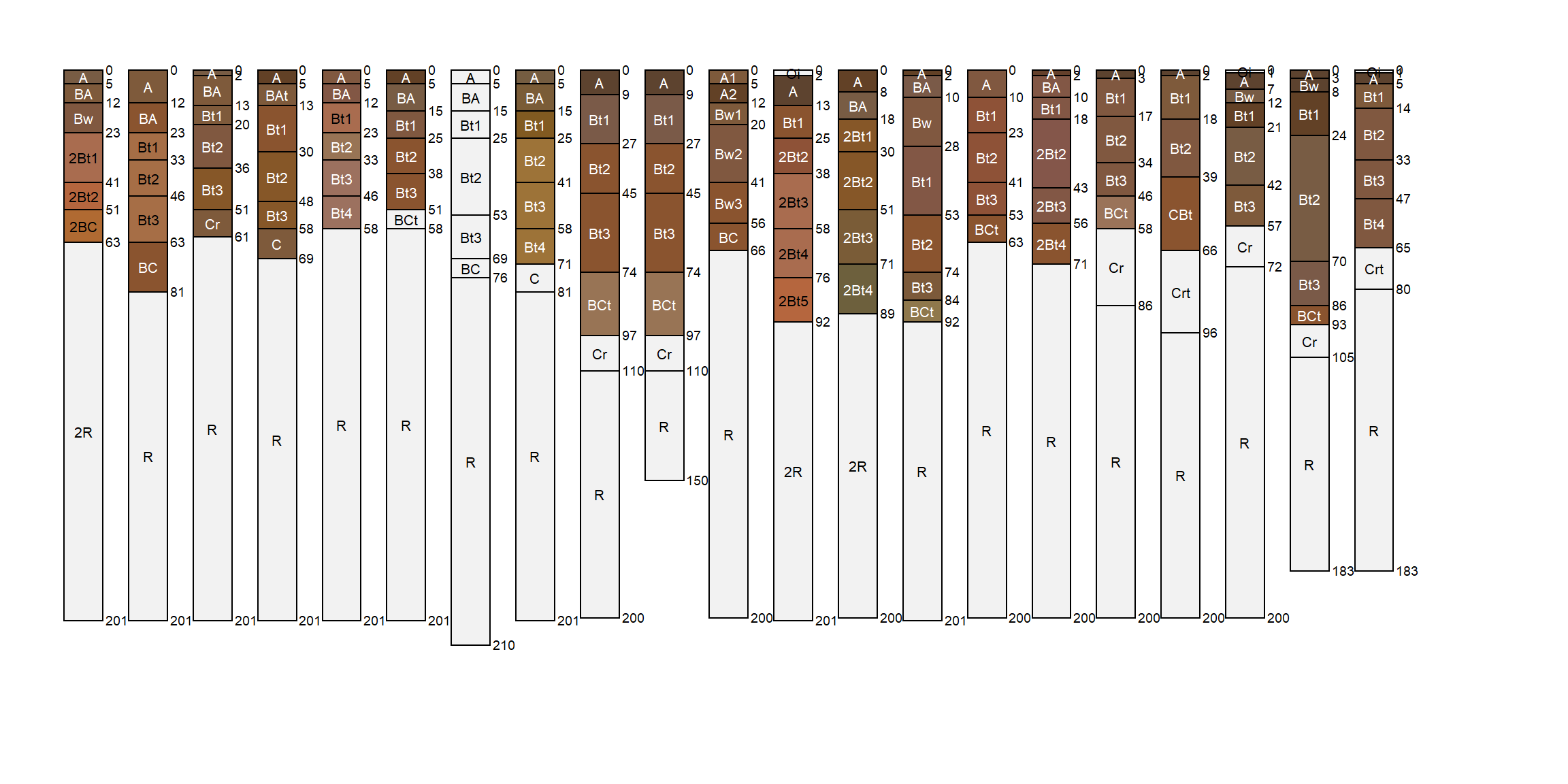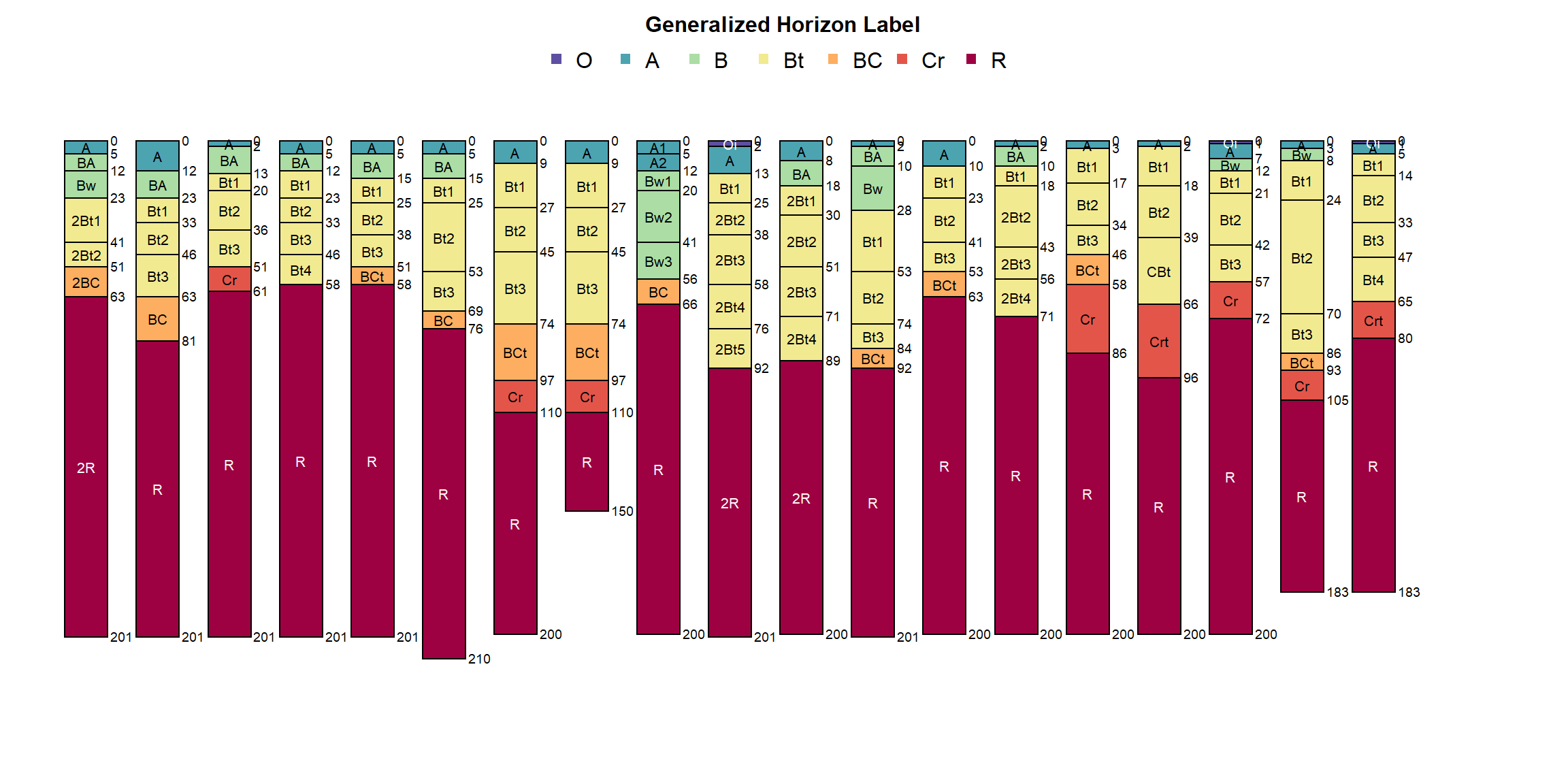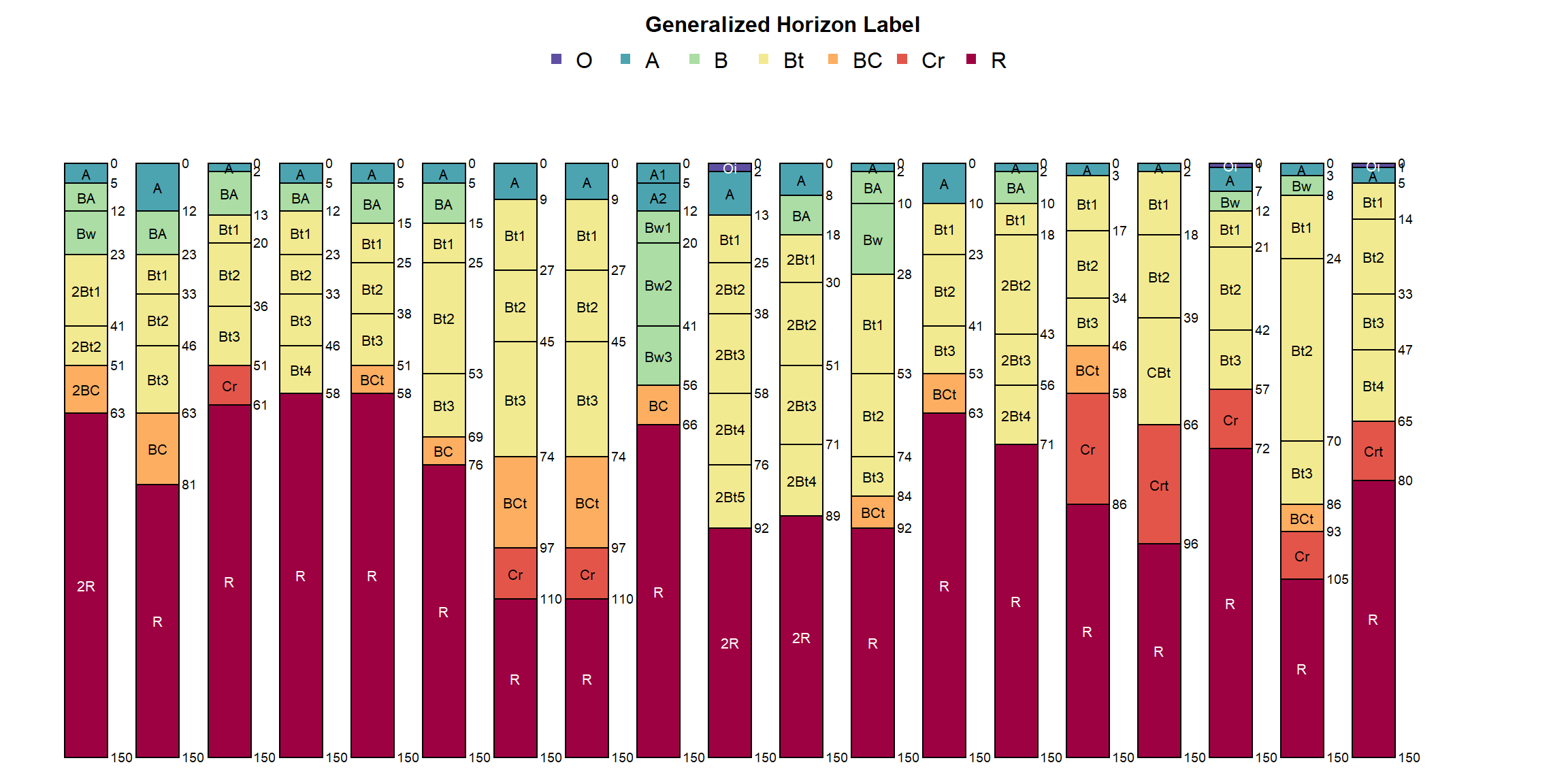Question: is it possible to generate a reasonable sorting of soil profiles (a `SoilProfileCollection`) using pair-wise distances computed only from generalized horizon labels (GHL)? I think that it might, as long as these labels are encoded as ordered factors. We can use `profile_compare` to test this hypothesis. One might attempt such a comparison within a collection of similar soils where the only reliable data available are horizon depths and designations.

We will be using a subset of the the `loafercreek` sample data from the {soilDB} package.

``````# latest version from github
library(aqp)
library(soilDB)
library(sharpshootR)

library(cluster)
library(dendextend)
library(MASS)

data("loafercreek")

# profiles 20-40
x <- loafercreek[20:40, ]

# modify default arguments for some flair
par(mar = c(0, 0, 0, 0))
plotSPC(x, width = 0.3, name.style = 'center-center', plot.depth.axis = FALSE, hz.depths = TRUE, cex.names = 0.65, print.id = FALSE)``````Apply generalized horizon labels using overly-simplistic rules. This is only a demonstration.

``````# new labels
n <- c('O', 'A', 'B', 'Bt', 'BC', 'Cr', 'R')
# GHL patterns
p <- c('O', 'A', 'B', 'Bt', 'BC', 'Cr', 'R')

horizons(x)\$genhz <- generalize.hz(x\$hzname, new = n, pat = p)

# the most important step, genhz must be encoded as an ordered factor
x\$genhz <- ordered(x\$genhz)

# check frequency of GHL assignment
table(x\$genhz)``````
``````##
##        O        A        B       Bt       BC       Cr        R not-used
##        3       22       18       66       11        8       21        2``````

Remove profiles containing horizons assigned to the “not-used” GHL.

``````x\$flag <- profileApply(x, function(i) {
any(i\$genhz == 'not-used')
})

# SPC-aware version of subset()
y <- subset(x, !flag)

# check: ok
table(y\$genhz)``````
``````##
##        O        A        B       Bt       BC       Cr        R not-used
##        3       20       16       59       11        8       19        0``````
``````par(mar = c(0, 0, 3, 0))
plotSPC(y, color = 'genhz', width = 0.3, name.style = 'center-center', plot.depth.axis = FALSE, hz.depths = TRUE, cex.names = 0.65, col.label = 'Generalized Horizon Label', print.id = FALSE)``````Add empty horizons to bottom of the profiles with bottom-most horizon depth < 150cm, label with ‘R’ GHL. Truncate deeper profiles to 150cm. See `?fillHzGaps` and `?trunc` for details.

``````# extend with empty horizons
z <- fillHzGaps(y, to_top = NA, to_bottom = 150)

# label as 'R'
z\$hzname[is.na(z\$genhz)] <- 'R'
z\$genhz[is.na(z\$genhz)] <- 'R'

# truncate all profiles to 150cm
z <- trunc(z, z1 = 0, z2 = 150)

par(mar = c(0, 0, 3, 0))
plotSPC(z, color = 'genhz', width = 0.3, name.style = 'center-center', plot.depth.axis = FALSE, hz.depths = TRUE, cex.names = 0.65, col.label = 'Generalized Horizon Label', print.id = FALSE)``````Arrange profiles by depth to contact using `plot.order` argument to `plotSPC`. See `?getSoilDepthClass` and `?estimateSoilDepth` for details on estimating soil depth.

``````# add soil depth information to site
sdc <- getSoilDepthClass(z)
site(z) <- sdc

par(mar = c(0, 0, 3, 0))
plotSPC(z, color = 'genhz', width = 0.3, name.style = 'center-center', plot.depth.axis = FALSE, hz.depths = TRUE, cex.names = 0.65, plot.order = order(z\$depth), col.label = 'Generalized Horizon Label', print.id = FALSE)``````